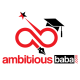RRB NTPC General intelligence & Reasoning quiz for Stage I : 05/03/2019

RRB NTPC General intelligence & Reasoning quiz for Stage I

General intelligence & Reasoning quiz for RRB NTPC, RRB JE(phase I) and other railways exam, and other competitive exams.

Q1. In the following question, select the related word pair from the given alternatives.

Sand : Desert : : ? : ?

A) Ocean : Water

B) Pond : Water

C) Eat : Smoke

D) Water : Sea

Q2.  In the following question, select the related number pair from the given alternatives.

33 : 38 : : ? : ?

A) 17 : 24

B) 12 : 18

C) 10 : 20

D) 13 : 18

Q3.  In the following question, select the related letter/letters from the given alternatives.

ORS : NQR : : GHK : ?

A) FGJ

B) FIL

C)  HIL

D) HIM

Q4.  In the following question, four number pairs are given. The number on left side of (–) is related to the number on the right side of (–) with some Logic/Rule/Relation. Three are similar on basis of same Logic/Rule/Relation. Select the odd one out from the given options.

A) 15 – 12

B) 21 – 18

C) 25 – 22

D) 27 – 22

Q5. From the given alternatives, according to dictionary, which word will come at THIRD position?
1. Massive
2. Metal
3. Method
4. Measure
5. Match

A) Massive

B) Measure
C) Method
D) Match

Q6. In the following question, select the missing number from the given series.

13.5, 14.5, 31, 128, ?

A) 1032

B) 1096

C) 1164

D) 844

Q7.  In a row of boys, Rahul is 31st from top and Vinod is 53rd from bottom. There are 22 boys between Vinod and Rahul. There are 15 girls in a other row. How many minimum students are there?

A) 65

B) 72

C) 75

D) 80

Q8. From the given alternatives, select the word which CANNOT be formed using the letters of the given word.

Misinformative

A) Form

B) Motive

C) Inform

D) Many

Q9. In a certain code language, “FUMES” is written as “62113519” and “MONTE” is written as “131514205”. How is “CARLO” written in that code language?

A) 31181215

B) 4181256
C)  4111185

D)  21812165

Q10.  In a certain code language, ‘-‘ represents ‘+’, ‘+’ represents ‘x’, ‘x’ represents ‘÷’ and ‘÷’ represents ‘-‘. Find out the answer to the following question.

10 ÷ 4 – 10 + 6 x 2 = ?

A) 39

B) 11

C) 21

D) 36

Solutions

Q1. Ans(D)

Desert is made up of abundance of sand similarly sea is made up of abundance of water.

Q2. Ans(D)

3 + 5 = 38
Similarly, 13 + 5 = 18

Q3. Ans(A)

-1 series

Q4. Ans(D)

-3 series except option D

Q5. Ans(B)

Massive – match – measure – metal – method
Clearly, measure is in third position.

Q6. Ans(A)

(13.5+ 1) × 1 = 14.5
(14.5 + 1) × 2 = 31, (31 + 1)× 4 = 128
(128+1) × 8 = 1032

Q7. Ans(C)Q8. Ans(D)

Many can’t be formed because y is not available in given word.

Q9. Ans(A)Q10. Ans(D)

10 ÷ 4 – 10 + 6 × 2
After changing sign
10 – 4 + 10 × 6 ÷ 2
= 40 – 4
= 36

WhatsApp Group Join here

Mail us at : ambitiousbaba1@gmail.com

•Ambitious Baba